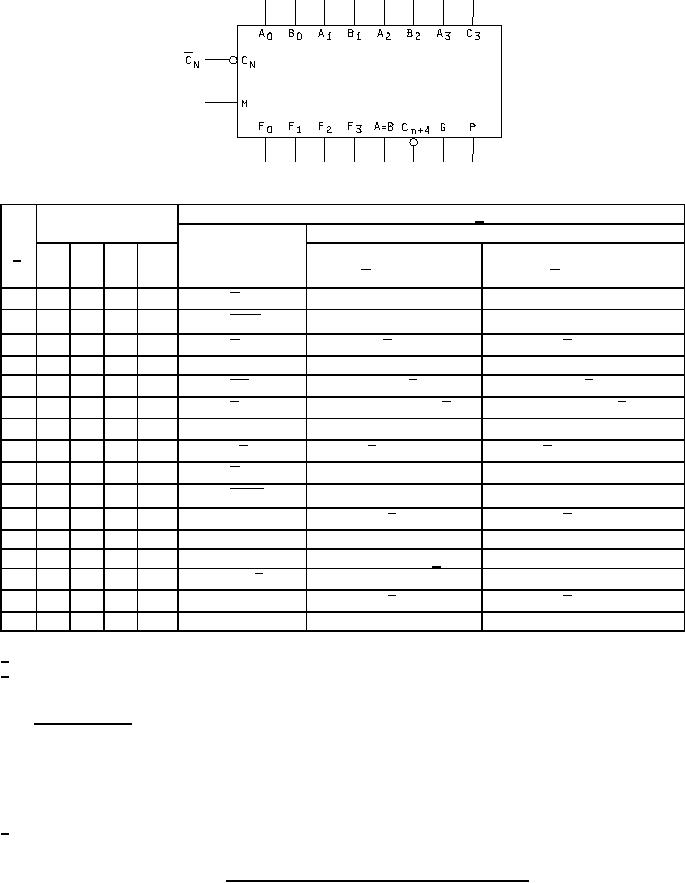Click here to make tpub.com your Home Page Page Title: Figure 2. Truth tables and logic equations for device type 01. Back | Up | NextWeb www.tpub.comHome

Information Categories
Aerographer
Automotive
Aviation
Construction
Diving
Draftsman
Engineering
Electronics
Food and Cooking
Logistics
Math
Medical
Music
Nuclear Fundamentals
Photography
ReligionMIL-M-38510/308B
Active high data 3/
Selection
M=H
M = L; Arithmetic operations
2/
Logic functions
Cn = 0
Cn = 1
S3
S2
S1
S0
Cn = 1 =H
Cn = 0 =H
0
L
L
L
L
F=A
F = A plus 1
F= A
F = A +B
F=A+B
F = (A + B) plus 1
1
L
L
L
H
2
L
L
H
L
F= AB
F=A+ B
F = (A + B ) plus 1
3
L
L
H
H
F=0
F = minus 1 (2's compl)
F = zero
4
L
H
L
L
F = AB
F = A plus A B
F = A plus A B plus 1
5
L
H
L
H
F= B
F = (A + B) plus A B
F = (A + B) plus A B plus 1
F = AB
6
L
H
H
L
F = A minus B minus 1
F = A minus B
7
L
H
H
H
F = AB
F = A B minus 1
F = AB
8
H
L
L
L
F = A plus AB
F = A plus AB plus 1
F= A +B
F = A B
F = A plus B
F = A plus B plus 1
9
H
L
L
H
10
H
L
H
L
F=B
F = (A + B ) plus AB
F = (A + B ) plus AB plus 1
11
H
L
H
H
F = AB
F = AB minus 1
F = AB
12
H
H
L
L
F=1
F = A plus A
1/
F = A plus A plus 1
13
H
H
L
H
F = (A + B) plus A
F = (A + B) plus A plus 1
F=A+ B
14
H
H
H
L
F=A+B
F = (A + B ) plus A
F = (A + B ) plus A plus 1
15
H
H
H
H
F=A
F = A minus 1
F=A
1/ Each bit is shifted to the next more significant position.
2/ This device (ALU) can be used as a comparator when placed in the subtract mode (i. e., S3 S2 S1 S0 are at
logical levels LHHL respectively) and the following expressions are valid:
Active high data
When Cn is high and Cn+4 is high, then A B
When Cn is low and Cn+4 is high, then A < B
When Cn is high and Cn+4 is low, then A > B
When Cn is low and Cn+4 is low, then A B
3/ The table shown applies for positive logic. If negative logic is used, active high data becomes active low data.
FIGURE 2. Truth tables and logic equations for device type 01.
9Integrated Publishing, Inc. - A (SDVOSB) Service Disabled Veteran Owned Small Business